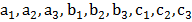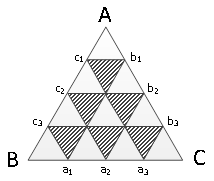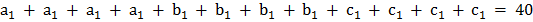### Sample Problem

The perimeter of triangle ABC is 40 cm. Ifdivide BC, AC and AB into 4 equal parts, then what is the perimeter of the shaded region?

cm#### Solution

We see that each shaded triangle have a perimeter of.

We know thatSo= 40 ÷ 4 = 10.

We are looking for the perimeter of

6,

which is simply 10 × 6 = 60 cm.